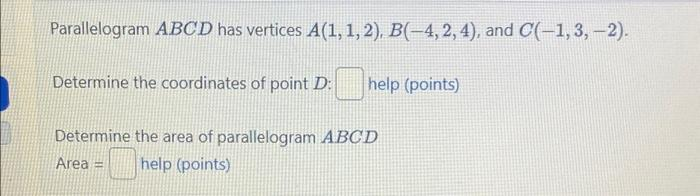# (Solved): Parallelogram ABCD has vertices A(1, 1, 2), B(4, 2, 4), and C(-1, 3, -2). Determine the coordinat ...

Parallelogram ABCD has vertices A(1, 1, 2), B(–4, 2, 4), and C(-1, 3, -2). Determine the coordinates of point D: help (points) Determine the area of parallelogram ABCD Area = help (points)Parallelogram has vertices , and . Determine the coordinates of point : help (points) Determine the area of parallelogram Area help (points)

We have an Answer from Expert

Solution:

Parallelogram    has vetices    and

Let coordinate of    is

Let mid point of    and mid point of    is
Let coordinate of    is

Mid point of   :

Mid point of  :

We have an Answer from Expert